If you have a related question, please click the "Ask a related question" button in the top right corner. The newly created question will be automatically linked to this question.

BQ20Z80A-V110: Need to know the specific way that the 4 byte hexadecimal value is calculated for floating point values in the bq20z80a-v110 TI chip

Part Number: BQ20Z80A-V110
Other Parts Discussed in Thread: BQ29312A

To whom it may concern, I am attempting to convert a floating pointnumber to its equivalent hexadecimal value in floating point notation in 4 size bytes, per the document, bq20z80A-V110 + bq29312A Chipset Technical Reference Manual  (Document  sluu288.pdf), in order to program the bq20z80a-v110   chip

I am using Labview in order to program the chip. The equation I am using is one to convert a floating point number to its IEEE-754 equivalent number.  The number initially programmed into the chip is .471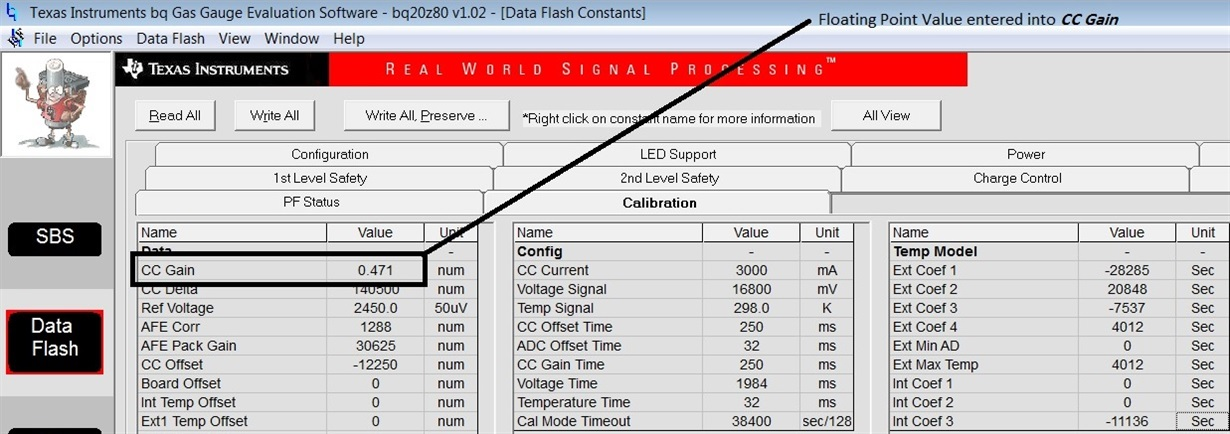When I check the 4 byte hex value that is programmed into the chip for this number, it is 7F 71 26 E9.

Now, if you check this value in an IEEE-754 online converter, the value for that 32 bit word is different than the one calculated from the bq evaluation software.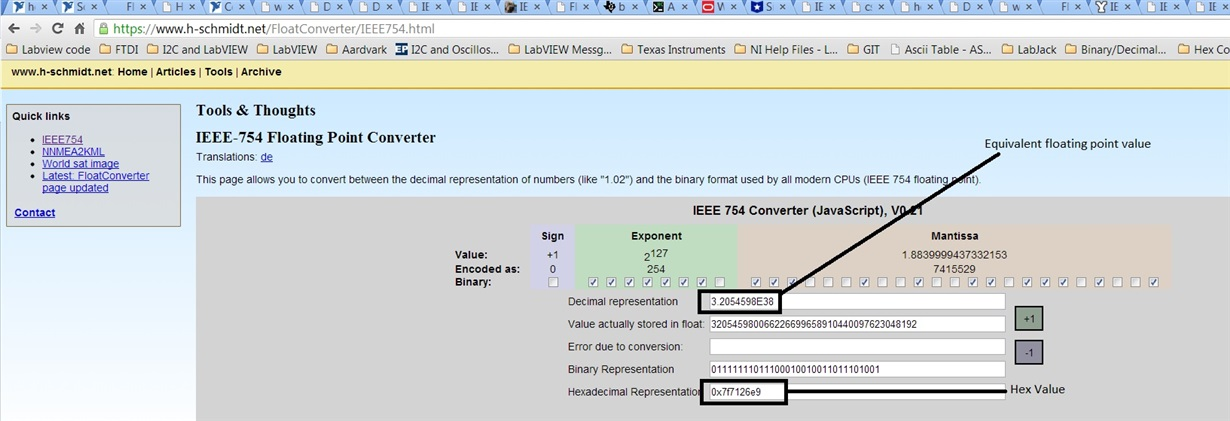Now, if I post the .471 value into the online converter, I get the following hexadecimal representation, 0x3ef126e9: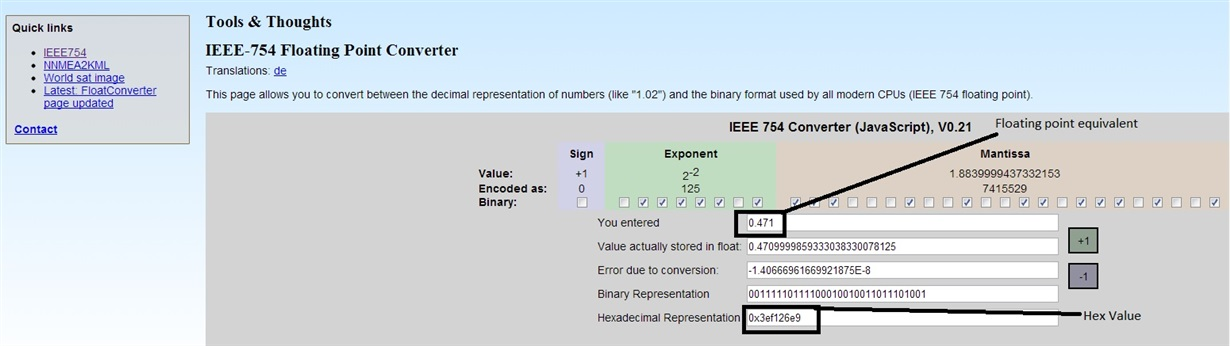As a check, I used another independent IEEE-754 calculator and got the same 4 byte hex value for the floating point number .471: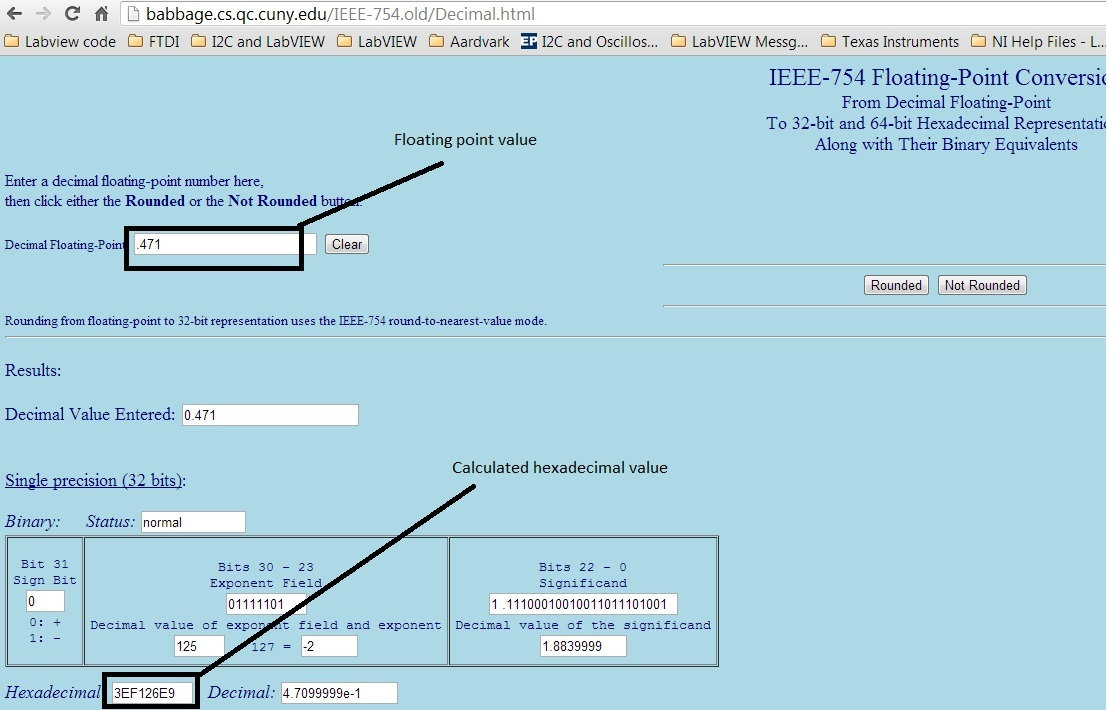Also, this is the value that I was able to generate from my Labview code, which was the same as the calculated value from the online calculations.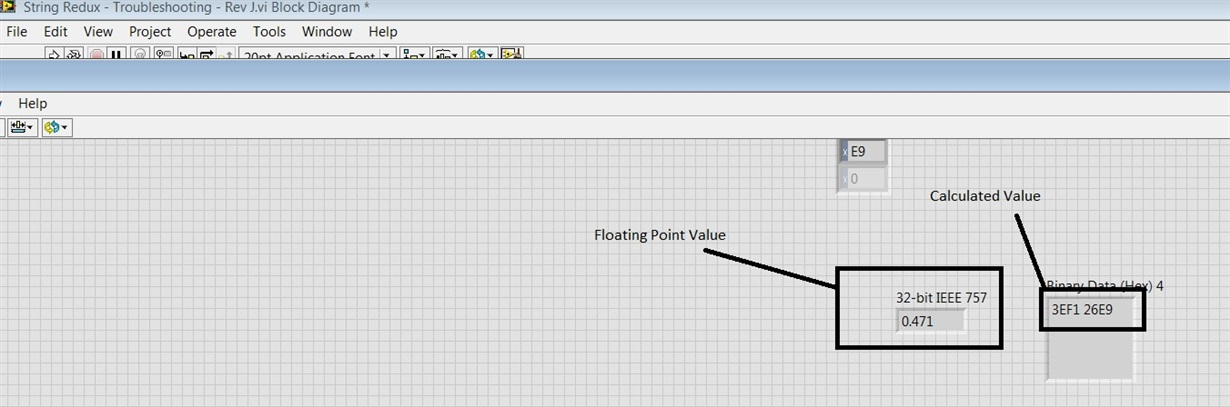Therefore, as it seems as if the industry standard IEEE-754 isn’t used by Texas Instruments for this chip, I would like to know what are the exact calculations for the floating point, 32-bit values that are used to translate between the aforementioned floating point values and the equivalent hex values.